# William Stallings Data and Computer Communications Chapter 5

• Slides: 63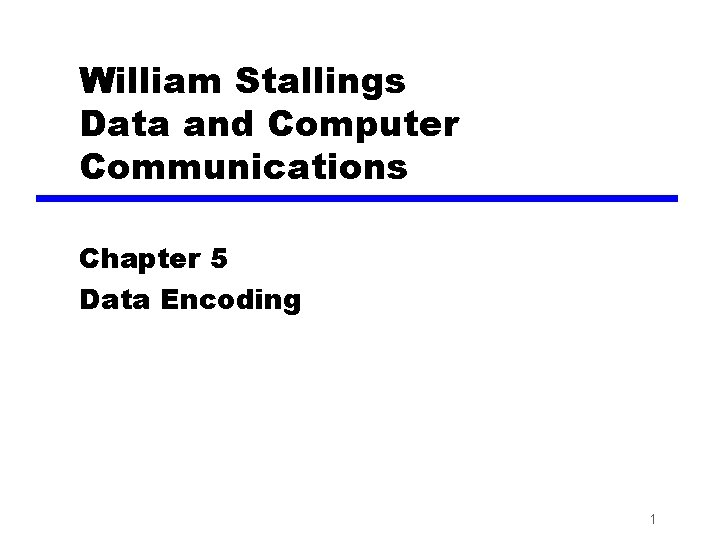William Stallings Data and Computer Communications Chapter 5 Data Encoding 1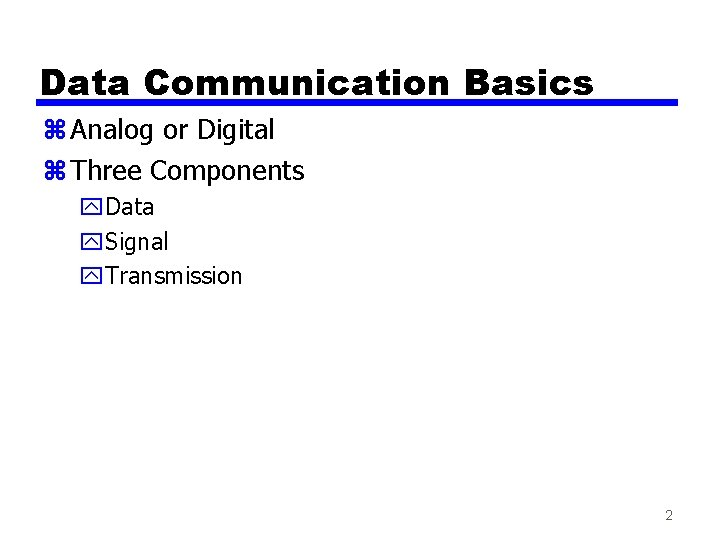Data Communication Basics z Analog or Digital z Three Components y. Data y. Signal y. Transmission 2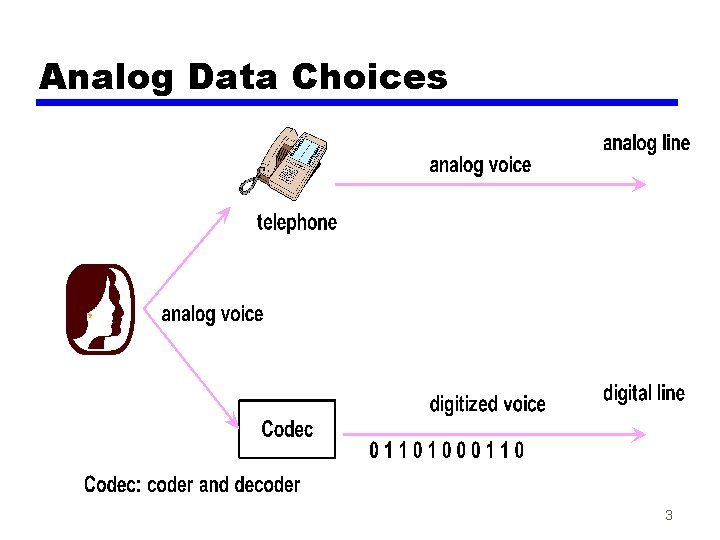Analog Data Choices 3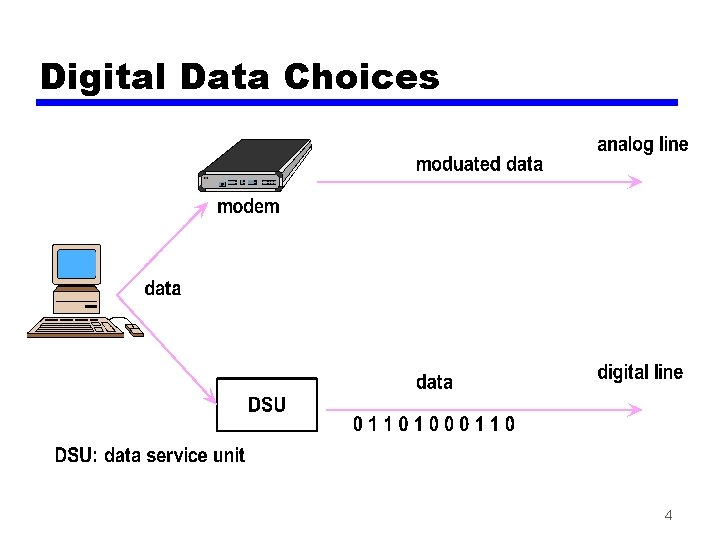Digital Data Choices 4Encoding Techniques z Digital data, digital signal z Analog data, digital signal z Digital data, analog signal z Analog data, analog signal 5Transmission Choices z Analog transmission yonly transmits analog signals, without regard for data content yattenuation overcome with amplifiers z Digital transmission ytransmits analog or digital signals yuses repeaters rather than amplifiers 6Advantages of Digital Transmission z The signal is exact z Signals can be checked for errors z Noise/interference are easily filtered out z A variety of services can be offered over one line z Higher bandwidth is possible with data compression 7Encoding schemes Analog data, Analog signal voice Telephone analog Digital data, Analog signal digital Modem analog Analog data, Digital signal analog digital CODEC Digital data, Digital signal digital Digital transmitter digital 8Encoding and Modulation x(t) g(t) digital or analog Encoder digital g(t) x(t) Decoder t s(f) s(t) m(t) Modulator digital or analog fc m(t) Demodulator f fc 9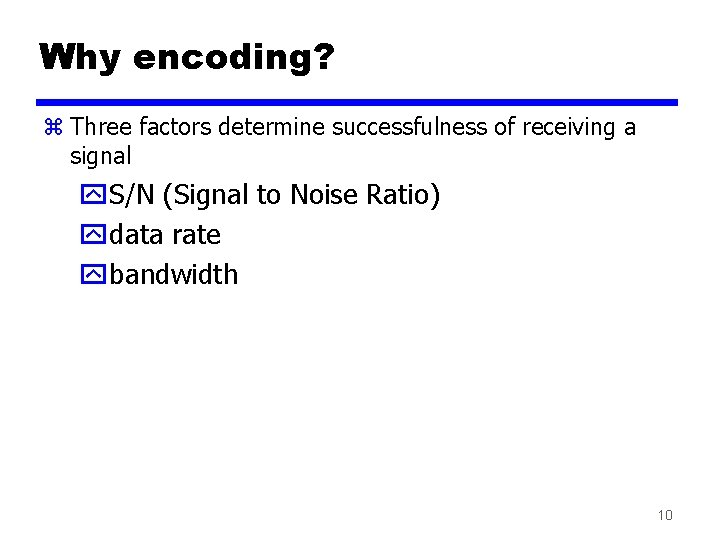Why encoding? z Three factors determine successfulness of receiving a signal y. S/N (Signal to Noise Ratio) ydata rate ybandwidth 10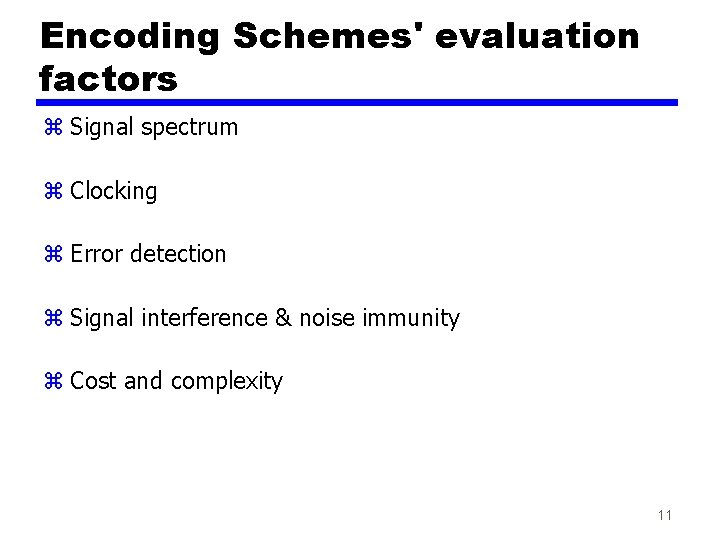Encoding Schemes' evaluation factors z Signal spectrum z Clocking z Error detection z Signal interference & noise immunity z Cost and complexity 11Digital Data, Digital Signal / Characteristics z Digital signal y. Uses discrete, discontinuous, voltage pulses y. Each pulse is a signal element y. Binary data is encoded into signal elements 12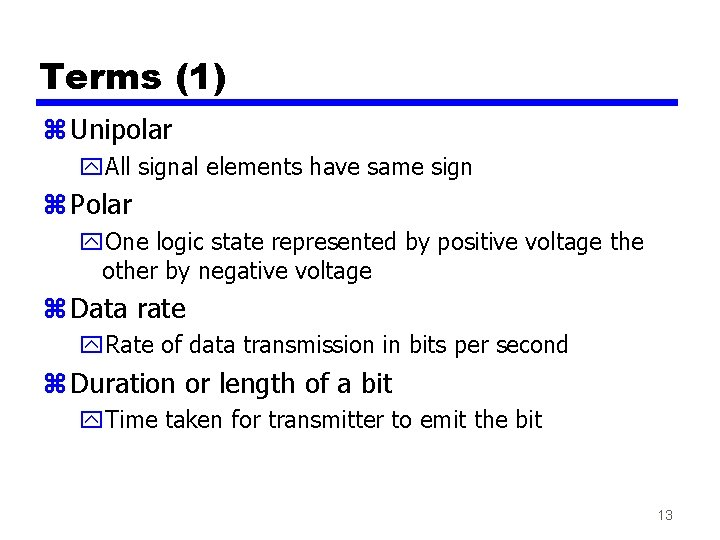Terms (1) z Unipolar y. All signal elements have same sign z Polar y. One logic state represented by positive voltage the other by negative voltage z Data rate y. Rate of data transmission in bits per second z Duration or length of a bit y. Time taken for transmitter to emit the bit 13Terms (2) z Modulation rate y. Rate at which the signal level changes y. Measured in baud = signal elements per second z Mark and Space y. Binary 1 and Binary 0 respectively 14Interpreting Signals z Need to know y. Timing of bits - when they start and end y. Signal levels z Factors affecting successful interpretation of signals: y. Signal to noise ratio y. Data rate y. Bandwidth 15Comparison of Encoding Schemes (1) z Signal Spectrum y. Lack of high frequencies reduces required bandwidth y. Lack of dc component allows ac coupling via transformer, providing isolation y. It is important to concentrate power in the middle of the bandwidth z Clocking issues y. Synchronizing transmitter and receiver is essential y. External clock is one way used for synchronization y. Synchronizing mechanism based on signal is also used & preferred (over using an external clock) 16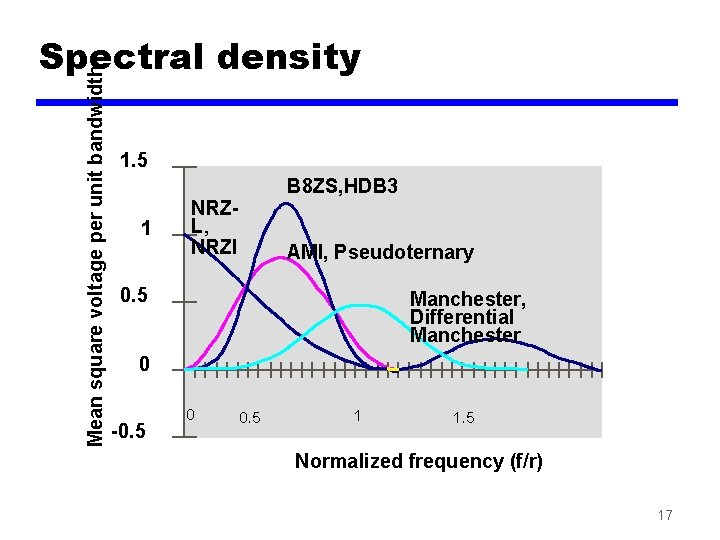Mean square voltage per unit bandwidth Spectral density 1. 5 1 B 8 ZS, HDB 3 NRZL, NRZI AMI, Pseudoternary 0. 5 Manchester, Differential Manchester 0 -0. 5 0 0. 5 1 1. 5 Normalized frequency (f/r) 17Comparison of Encoding Schemes (2) z Error detection y. Can be built into signal encoding z Signal interference and noise immunity y. Some codes are better than others z Cost and complexity y. Higher signal rate (& thus data rate) lead to higher costs y. Some codes require signal rate greater than data rate 18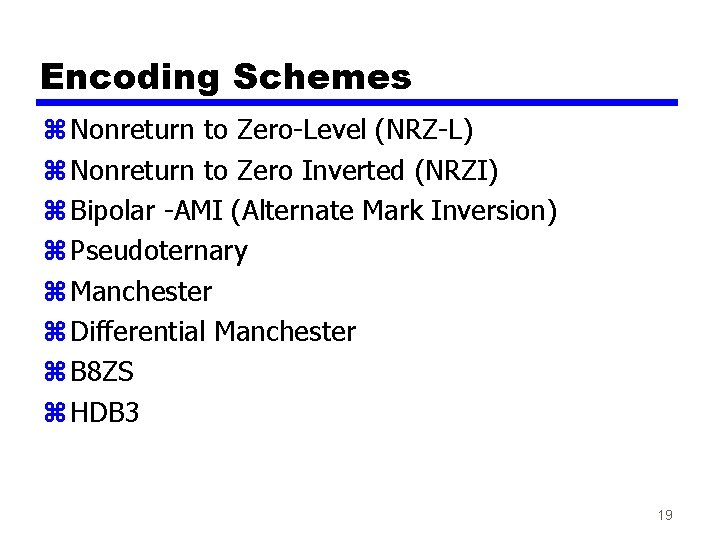Encoding Schemes z Nonreturn to Zero-Level (NRZ-L) z Nonreturn to Zero Inverted (NRZI) z Bipolar -AMI (Alternate Mark Inversion) z Pseudoternary z Manchester z Differential Manchester z B 8 ZS z HDB 3 19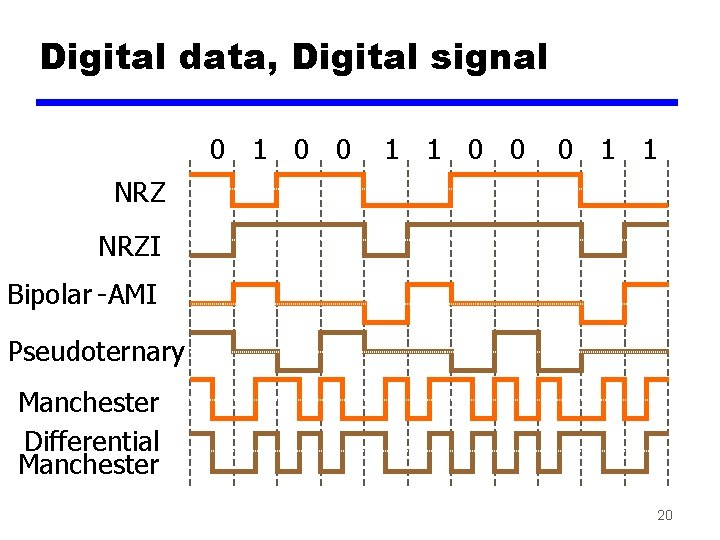Digital data, Digital signal 0 1 0 0 1 1 0 0 0 1 1 NRZI Bipolar -AMI Pseudoternary Manchester Differential Manchester 20Nonreturn to Zero-Level (NRZ-L) z Two different voltages for 0 and 1 bits z Voltage constant during bit interval z Most often, negative voltage for one value and positive for the other 21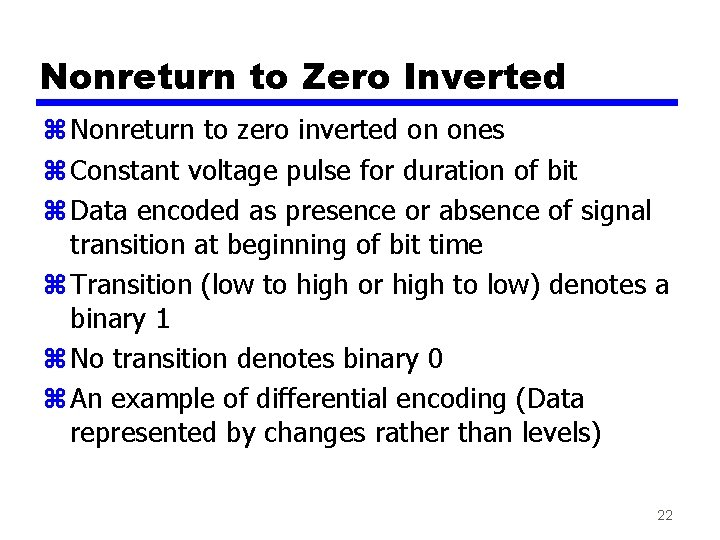Nonreturn to Zero Inverted z Nonreturn to zero inverted on ones z Constant voltage pulse for duration of bit z Data encoded as presence or absence of signal transition at beginning of bit time z Transition (low to high or high to low) denotes a binary 1 z No transition denotes binary 0 z An example of differential encoding (Data represented by changes rather than levels) 22NRZ 23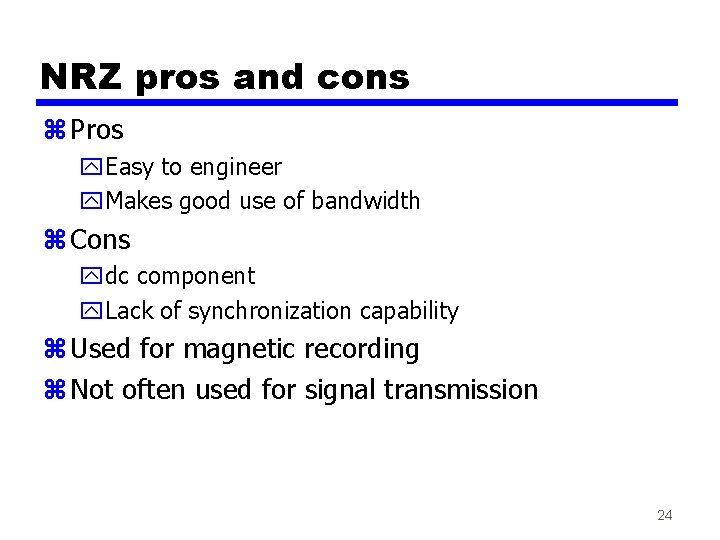NRZ pros and cons z Pros y. Easy to engineer y. Makes good use of bandwidth z Cons ydc component y. Lack of synchronization capability z Used for magnetic recording z Not often used for signal transmission 24Multilevel Binary z Use more than two levels z Bipolar-AMI yzero represented by no line signal yone represented by positive or negative pulse yone pulses alternate in polarity y. No loss of sync if a long string of ones happens (zeros still a problem) y. No net dc component Can use a transformer for isolating transmission line y. Lower bandwidth y. Easy error detection 25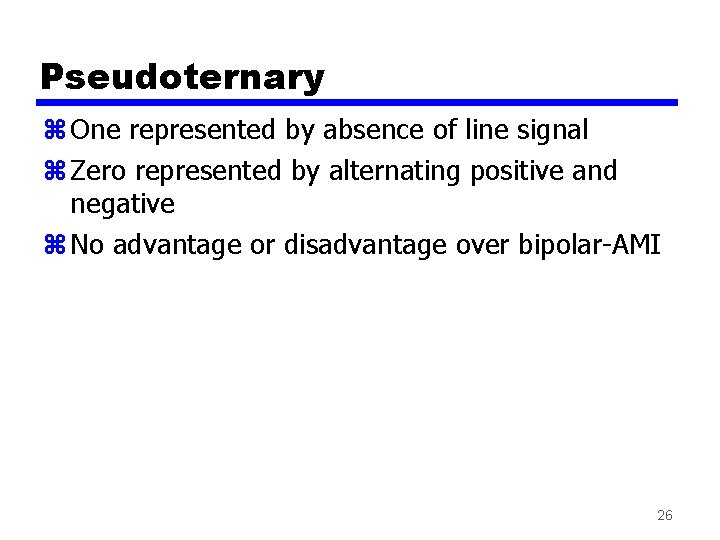Pseudoternary z One represented by absence of line signal z Zero represented by alternating positive and negative z No advantage or disadvantage over bipolar-AMI 26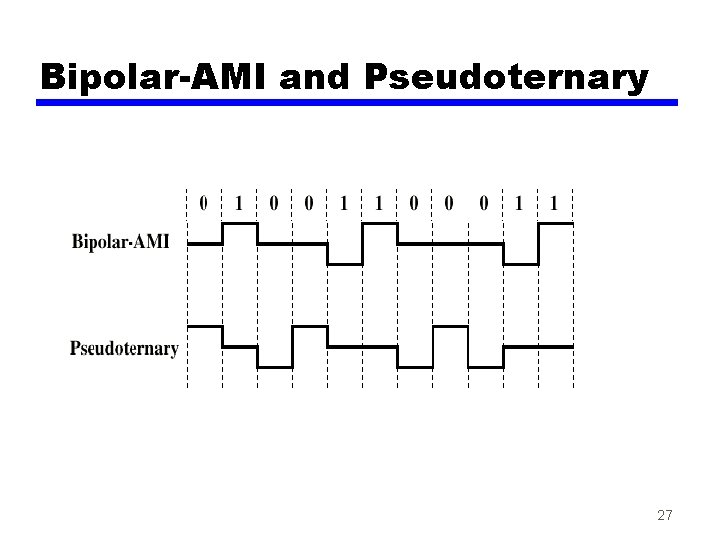Bipolar-AMI and Pseudoternary 27Trade Off for Multilevel Binary z Not as efficient as NRZ y. With multi-level binary coding, the line signal may take on one of 3 levels, but each signal element, which could represent log 23 = 1. 58 bits of information, bears only one bit of information y. Receiver must distinguish between three levels (+A, -A, 0) y. Requires approx. 3 d. B more signal power for same probability of bit error 28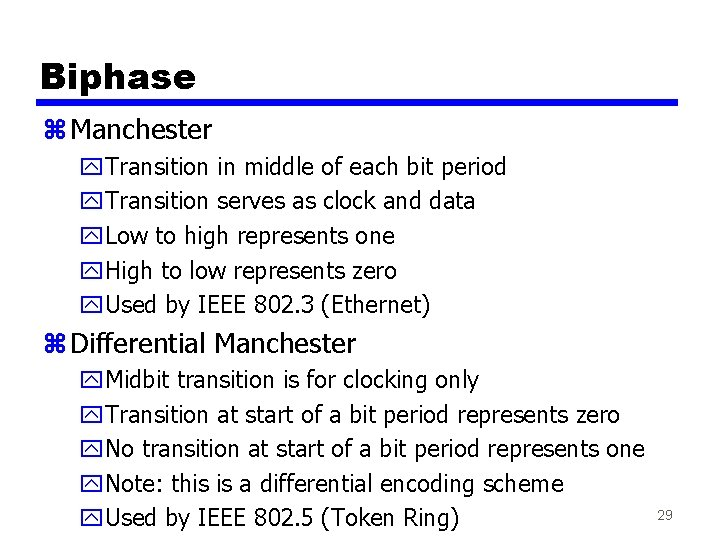Biphase z Manchester y. Transition in middle of each bit period y. Transition serves as clock and data y. Low to high represents one y. High to low represents zero y. Used by IEEE 802. 3 (Ethernet) z Differential Manchester y. Midbit transition is for clocking only y. Transition at start of a bit period represents zero y. No transition at start of a bit period represents one y. Note: this is a differential encoding scheme y. Used by IEEE 802. 5 (Token Ring) 29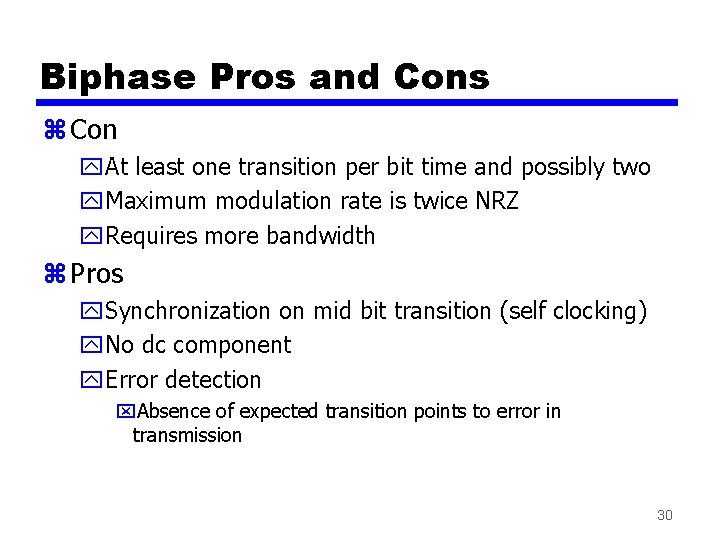Biphase Pros and Cons z Con y. At least one transition per bit time and possibly two y. Maximum modulation rate is twice NRZ y. Requires more bandwidth z Pros y. Synchronization on mid bit transition (self clocking) y. No dc component y. Error detection x. Absence of expected transition points to error in transmission 30Modulation Rate R=Data Rate=bits/sec=1 Mbps for both cases Modulation Rate=Baud Rate=Rate at which signal elements are generated=R for NRZI=2 R for Manchester 31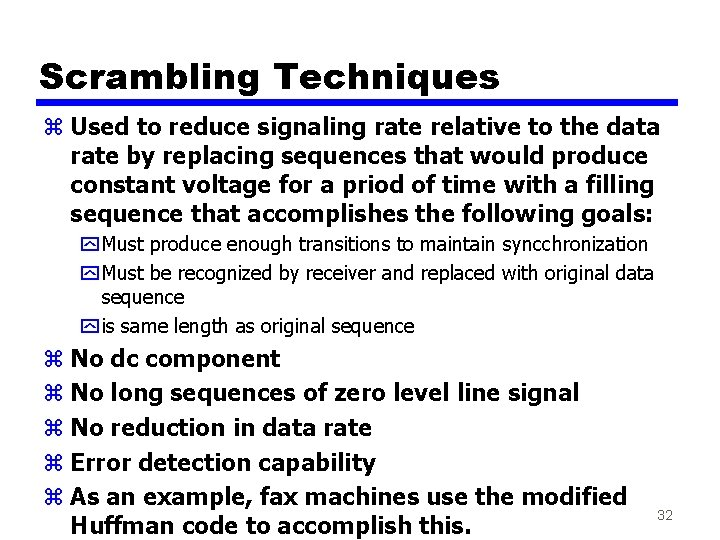Scrambling Techniques z Used to reduce signaling rate relative to the data rate by replacing sequences that would produce constant voltage for a priod of time with a filling sequence that accomplishes the following goals: y Must produce enough transitions to maintain syncchronization y Must be recognized by receiver and replaced with original data sequence y is same length as original sequence z No dc component z No long sequences of zero level line signal z No reduction in data rate z Error detection capability z As an example, fax machines use the modified Huffman code to accomplish this. 32B 8 ZS z Bipolar With 8 Zeros Substitution z Based on bipolar-AMI z If octet of all zeros and last voltage pulse preceding was positive, encode as 000+-0 -+ z If octet of all zeros and last voltage pulse preceding was negative, encode as 000 -+0+z Causes two violations of AMI code z This is unlikely to occur as a result of noise z Receiver detects and interprets the sequence as octet of all zeros 33HDB 3 z High Density Bipolar 3 Zeros z Based on bipolar-AMI z String of four zeros replaced with one or two pulses Note: The following is the explanation for the HDB 3 code example on the next slide (see rules in Table 5. 4, page 142): Assuming that an odd number of 1's have occurred since the last substitution, since the polarity of the preceding pulse is "-", then the first 4 zeros are replaced by "000 -". For the next 4 zeros, since there have been no Bipolar pulses since the 1 st substitution, then they are replaced by"+00+" since the preceding pulse is a "-". For the 3 rd case where 4 zeros happen, 2 (even) Bipolar pulses have happened since the last substitution and the polarity of the preceding pulse is "+", so "-00 -" is substituted for the zeros. 34B 8 ZS and HDB 3 (Assume odd number of 1 s since last substitution) See Table 5. 4 for HDB 3 Substitution Rules 35Digital Data, Analog Signal z Transmitting digital data through PSTN (Public telephone system) y 300 Hz to 3400 Hz bandwidth ymodem (modulator-demodulator) is used to convert digital data to analog signal and vice versa z Three basic modulation techniques are used: z Amplitude shift keying (ASK) z Frequency shift keying (FSK) z Phase shift keying (PSK) 36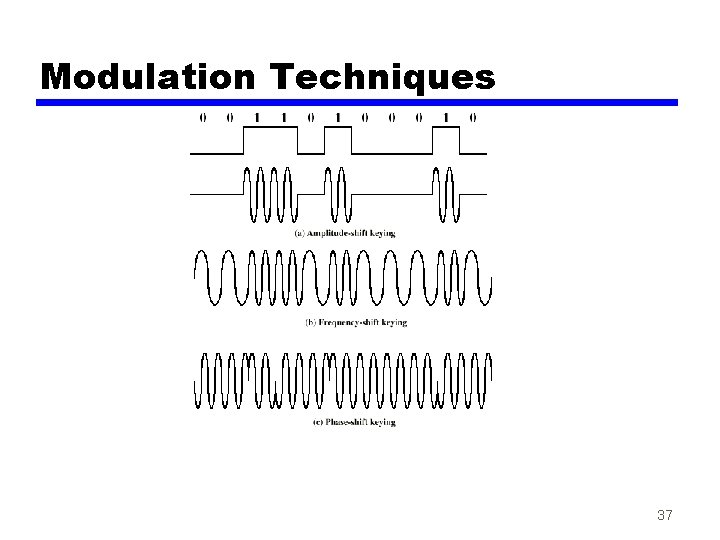Modulation Techniques 37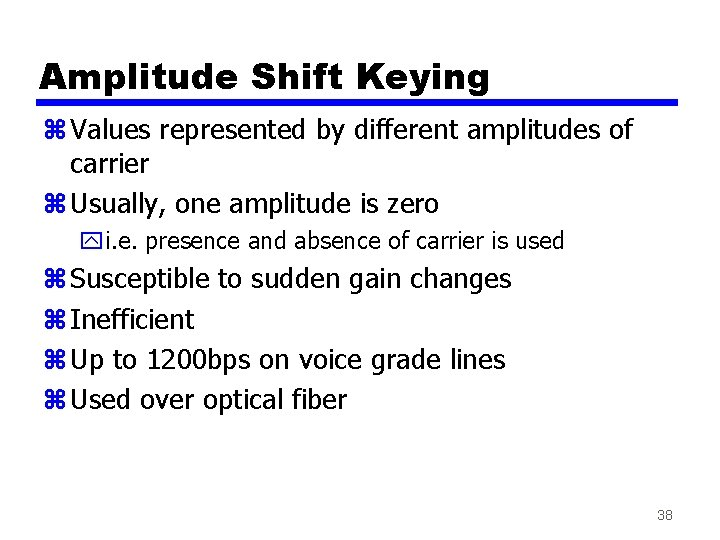Amplitude Shift Keying z Values represented by different amplitudes of carrier z Usually, one amplitude is zero yi. e. presence and absence of carrier is used z Susceptible to sudden gain changes z Inefficient z Up to 1200 bps on voice grade lines z Used over optical fiber 38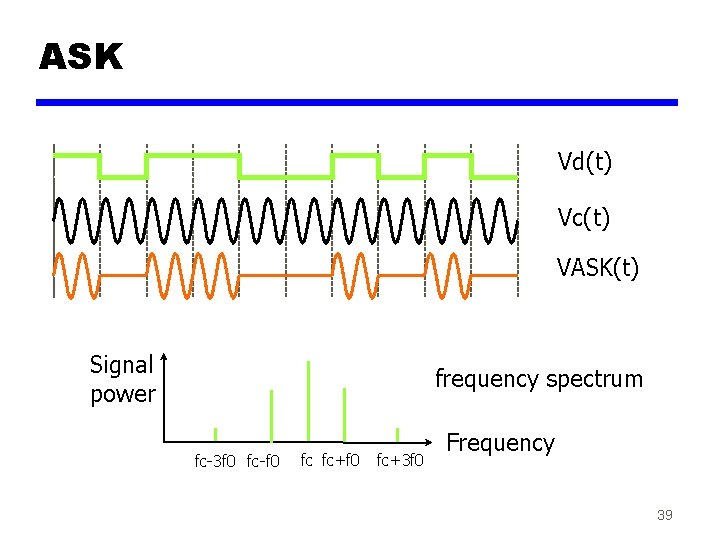ASK Vd(t) Vc(t) VASK(t) Signal power frequency spectrum fc-3 f 0 fc-f 0 fc fc+f 0 fc+3 f 0 Frequency 39Frequency Shift Keying z Values represented by different frequencies (near carrier) z Less susceptible to error than ASK z Up to 1200 bps on voice grade lines z High frequency radio (3 -30 MHz) z Higher frequency on LANs using co-ax 40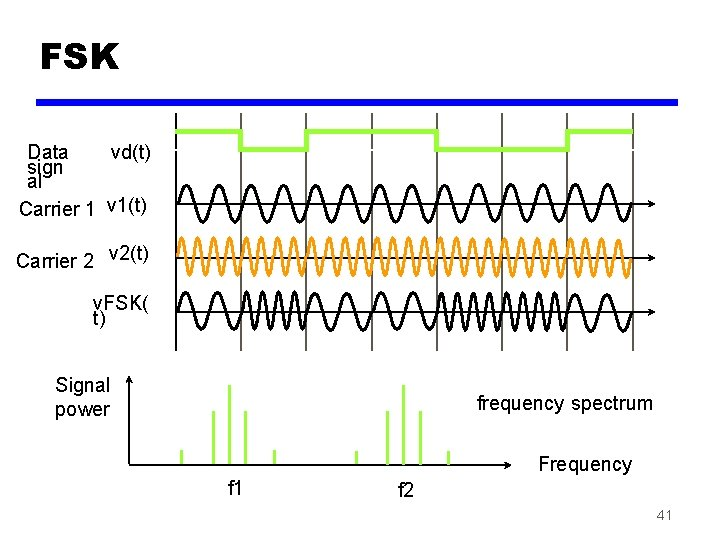FSK Data vd(t) sign al Carrier 1 v 1(t) Carrier 2 v 2(t) v. FSK( t) Signal power frequency spectrum Frequency f 1 f 2 41FSK in modem (on Voice Grade Line) Amplitude PSTN bandwidth 400 3400 Frequency(Hz) 1180 1650 (1270) (2025) 1850 980 (2225) (1070) frequency spectru 42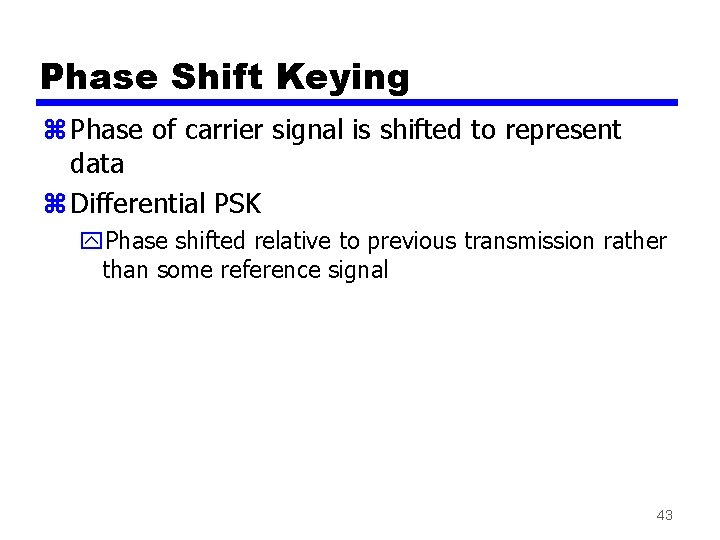Phase Shift Keying z Phase of carrier signal is shifted to represent data z Differential PSK y. Phase shifted relative to previous transmission rather than some reference signal 43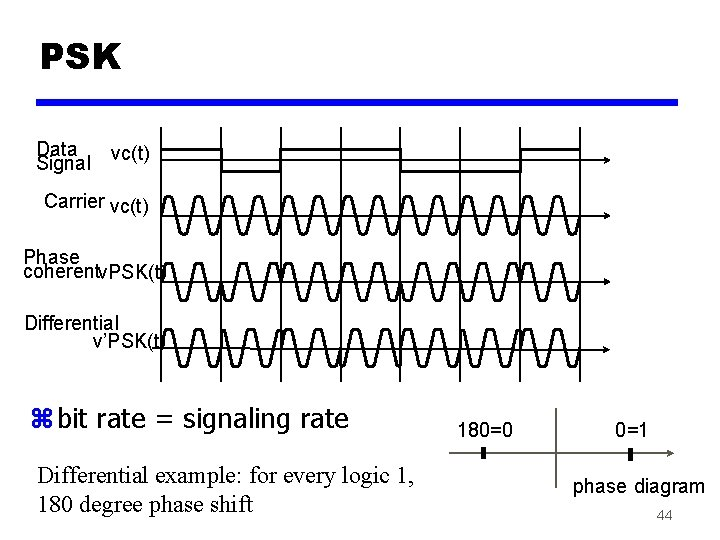PSK Data Signal vc(t) Carrier vc(t) Phase coherentv. PSK(t) Differential v’PSK(t) z bit rate = signaling rate Differential example: for every logic 1, 180 degree phase shift 180=0 0=1 phase diagram 44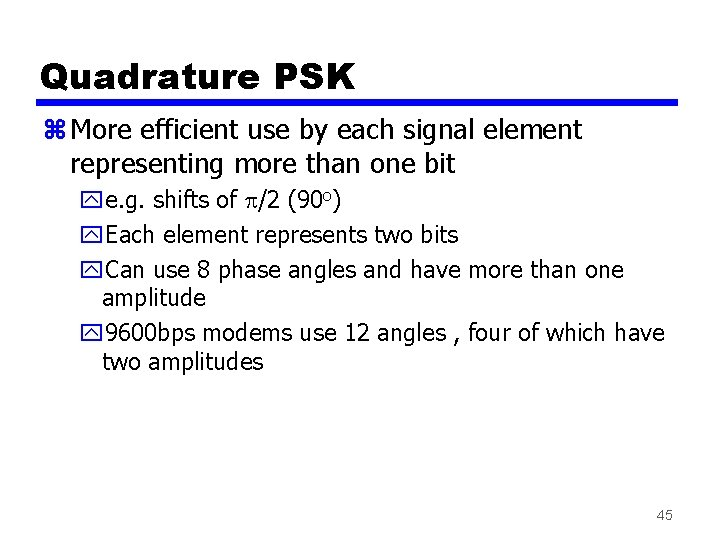Quadrature PSK z More efficient use by each signal element representing more than one bit ye. g. shifts of /2 (90 o) y. Each element represents two bits y. Can use 8 phase angles and have more than one amplitude y 9600 bps modems use 12 angles , four of which have two amplitudes 45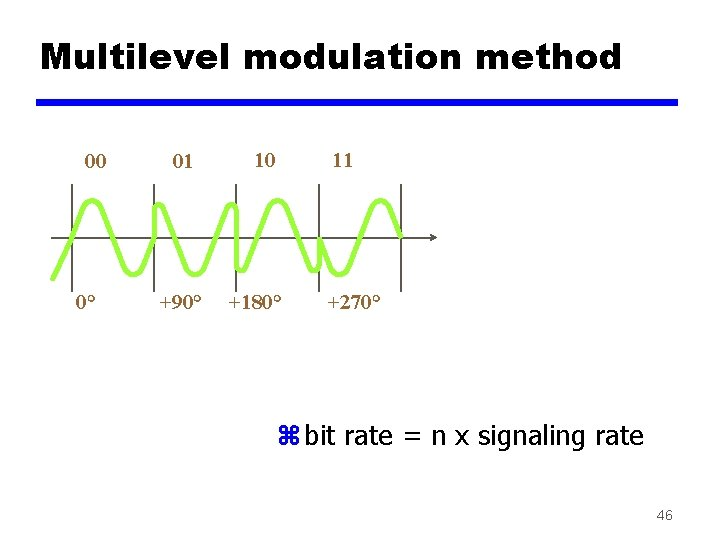Multilevel modulation method 00 0° 01 10 11 +90° +180° +270° z bit rate = n x signaling rate 46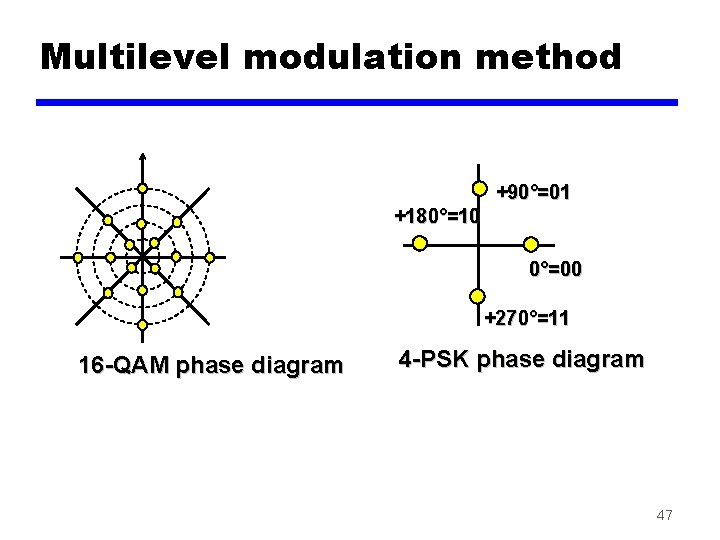Multilevel modulation method +90°=01 +180°=10 0°=00 +270°=11 16 -QAM phase diagram 4 -PSK phase diagram 47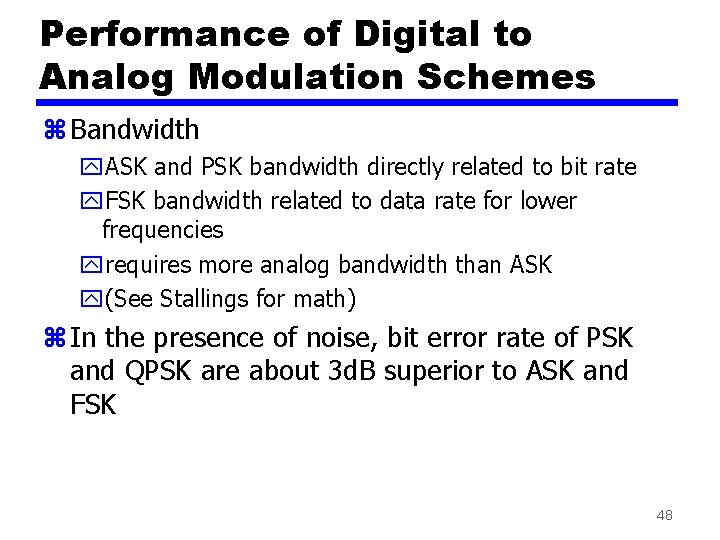Performance of Digital to Analog Modulation Schemes z Bandwidth y. ASK and PSK bandwidth directly related to bit rate y. FSK bandwidth related to data rate for lower frequencies yrequires more analog bandwidth than ASK y(See Stallings for math) z In the presence of noise, bit error rate of PSK and QPSK are about 3 d. B superior to ASK and FSK 48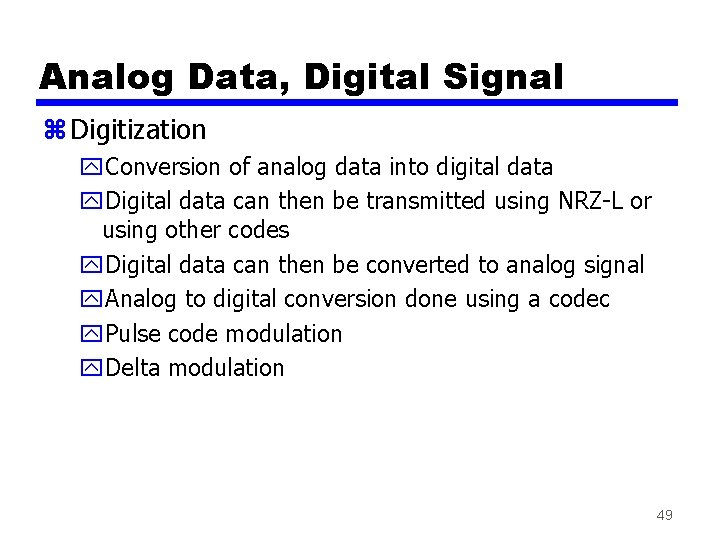Analog Data, Digital Signal z Digitization y. Conversion of analog data into digital data y. Digital data can then be transmitted using NRZ-L or using other codes y. Digital data can then be converted to analog signal y. Analog to digital conversion done using a codec y. Pulse code modulation y. Delta modulation 49Analog data, Digital signal z Two principle techniques used y. PCM (Pulse Code Modulation) y. DM (Delta Modulation) Sampling PAM signal clock Analog voice signal Sampling Circuit PCM signal Quantizer and compander Digitized voice signal 50Pulse Code Modulation(PCM) (1) z If a signal is sampled at regular intervals at a rate higher than twice the highest signal frequency, the samples contain all the information of the original signal y(Proof - Stallings appendix 4 A) z Voice data limited to below 4000 Hz z Require 8000 sample per second z Analog samples (Pulse Amplitude Modulation, PAM) z Each sample assigned digital value 51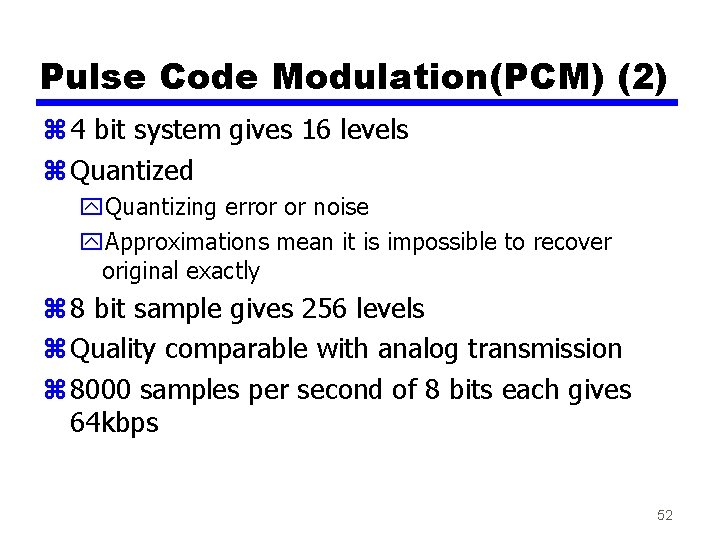Pulse Code Modulation(PCM) (2) z 4 bit system gives 16 levels z Quantized y. Quantizing error or noise y. Approximations mean it is impossible to recover original exactly z 8 bit sample gives 256 levels z Quality comparable with analog transmission z 8000 samples per second of 8 bits each gives 64 kbps 52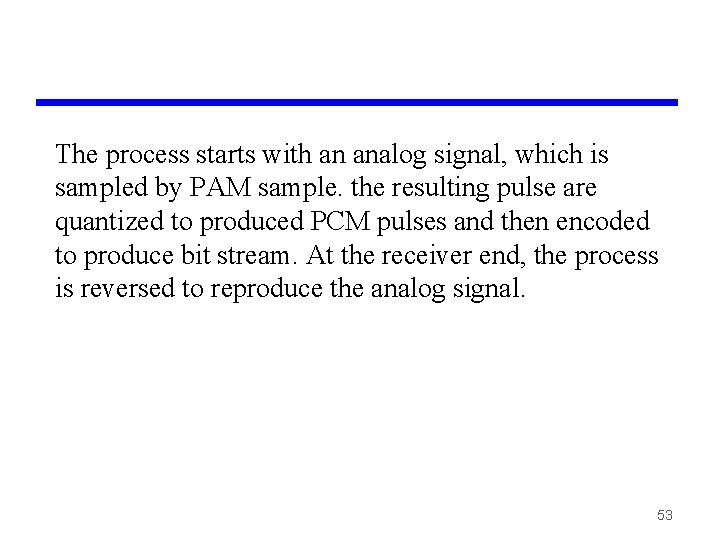The process starts with an analog signal, which is sampled by PAM sample. the resulting pulse are quantized to produced PCM pulses and then encoded to produce bit stream. At the receiver end, the process is reversed to reproduce the analog signal. 53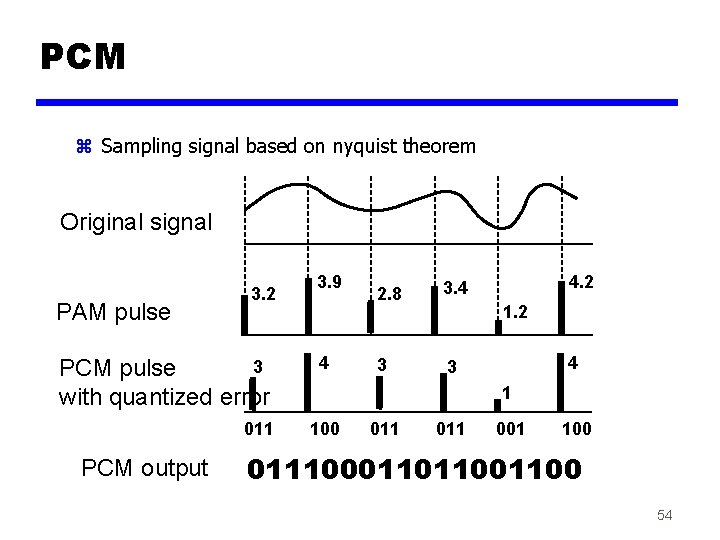PCM z Sampling signal based on nyquist theorem Original signal PAM pulse 3. 2 3 PCM pulse with quantized error 011 PCM output 3. 9 4 2. 8 3. 4 3 3 4. 2 1. 2 4 1 100 011 001 100 01110001101100 54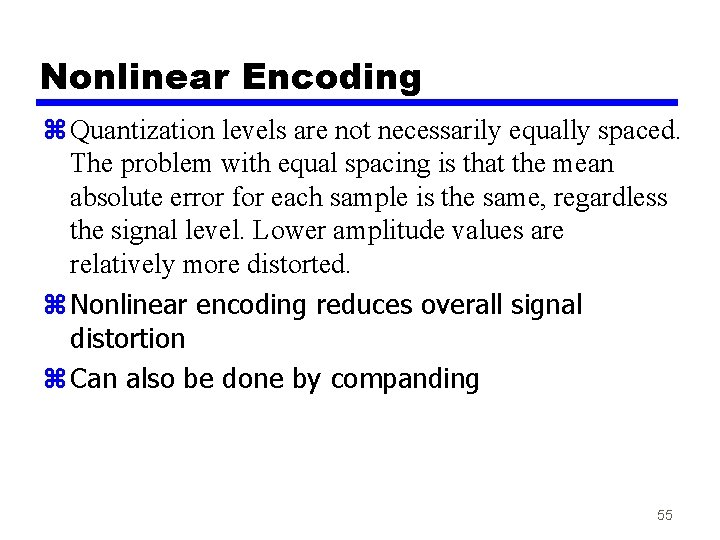Nonlinear Encoding z Quantization levels are not necessarily equally spaced. The problem with equal spacing is that the mean absolute error for each sample is the same, regardless the signal level. Lower amplitude values are relatively more distorted. z Nonlinear encoding reduces overall signal distortion z Can also be done by companding 55Nonlinear encoding 15 14 13 12 11 10 9 8 7 6 5 4 3 2 1 0 Quantizing level 15 14 13 Strong signal Weak signal 12 11 10 9 8 76 5 4 3 2 1 0 Without nonlinear encoding With nonlinear encoding 56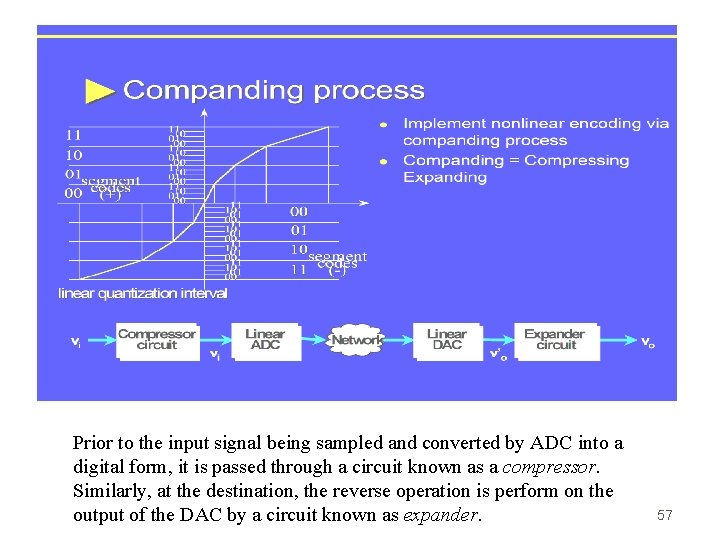Prior to the input signal being sampled and converted by ADC into a digital form, it is passed through a circuit known as a compressor. Similarly, at the destination, the reverse operation is perform on the output of the DAC by a circuit known as expander. 57Delta Modulation z Analog input is approximated by a staircase function z Move up or down one level ( ) at each sample interval z Binary behavior y. Function moves up or down at each sample interval 58Delta Modulation - example 59Delta Modulation - Performance z Good voice reproduction y. PCM - 128 levels (7 bit) y. Voice bandwidth 4 khz y. Should be 8000 x 7 = 56 kbps for PCM z Data compression can improve on this ye. g. Interframe coding techniques for video 60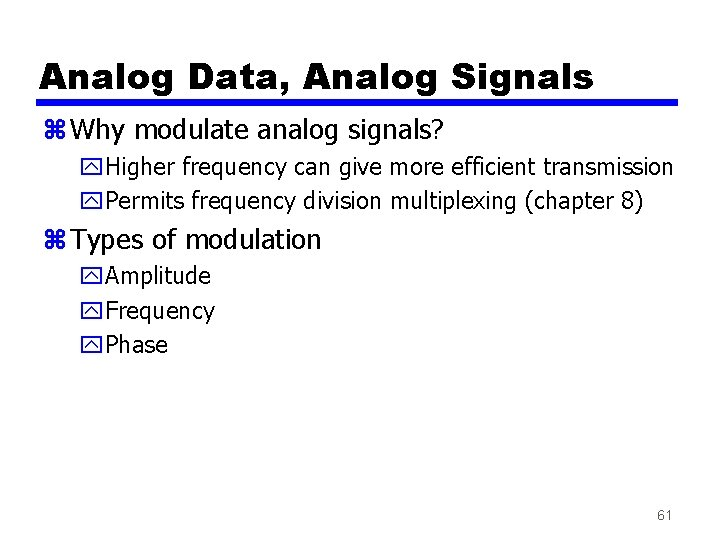Analog Data, Analog Signals z Why modulate analog signals? y. Higher frequency can give more efficient transmission y. Permits frequency division multiplexing (chapter 8) z Types of modulation y. Amplitude y. Frequency y. Phase 61Analog Modulation 62Spread Spectrum z Analog or digital data z Analog signal z Spread data over wide bandwidth z Makes jamming and interception harder z 2 schemes are used: z Frequency hoping y. Signal broadcast over seemingly random series of frequencies z Direct Sequence y. Each bit is represented by multiple bits in transmitted signal known as a chipping code 63